# An Example Using Boston House Prices Dataset¶

### Overview¶

For a long time I've been interested in TensorFlow. I've heard of the amazing things that people have achieved with this framework, and while I really wanted to dable in it, it seemed a daunting thing to install, let alone learn. The great news is that the Google brain team has made tremendous strides in making TensorFlow easy to install and use! Check out these earlier posts:

In this post we will see how easy it is to modify the regression tutorial published by the Google team to Boston House Prices data. We will learn:

• How to load a standard dataset from sklearn library into a data object (specifically, a data frame)
• How to explore and plot the data we just loaded
• How to split the data into training/testing
• How to further split the training data into calibration/validation so as to set a stopping criterion for neural net training
• How to fit a neural network model using Keras
• Keras is an open-source library written in python, and capable of running TensorFlow.
• How to make predictions using the model we trained
• How to analyze the error in the modeling

### Preliminaries¶

We need to make sure that a few relevant packages have been installed in this environment. If you recall, we created the 'tensorflow_env' environment within Anaconda, and this script is started from within that environment (via JupyterLab). We install the relevant packages below.

In :
!pip install -q seaborn

In :
pip install -U scikit-learn

Collecting scikit-learn
Collecting joblib>=0.11 (from scikit-learn)
Installing collected packages: joblib, scikit-learn
Successfully installed joblib-0.14.0 scikit-learn-0.21.3
Note: you may need to restart the kernel to use updated packages.


Initiate TensorFlow (tf), and load other libraries that we will need later (e.g. matplotlib, pandas, seaborn)

In :
from __future__ import absolute_import, division, print_function, unicode_literals

import pathlib

import matplotlib.pyplot as plt
import pandas as pd
import seaborn as sns

try:
# %tensorflow_version only exists in Colab.
%tensorflow_version 2.x
except Exception:
pass
import tensorflow as tf

from tensorflow import keras
from tensorflow.keras import layers

print(tf.__version__)

1.14.0


### Data exploration¶

First we import a the boston house prices dataset, and print a description of it so we can examine what is in the data. Remember in order to execute a 'cell' like the one below, you can 1) click on it and run it using the run button above or 2) click in the cell and hit shift+enter.

In :
import pandas as pd
print(boston.data.shape) #get (numer of rows, number of columns or 'features')
print(boston.DESCR) #get a description of the dataset

(506, 13)
.. _boston_dataset:

Boston house prices dataset
---------------------------

**Data Set Characteristics:**

:Number of Instances: 506

:Number of Attributes: 13 numeric/categorical predictive. Median Value (attribute 14) is usually the target.

:Attribute Information (in order):
- CRIM     per capita crime rate by town
- ZN       proportion of residential land zoned for lots over 25,000 sq.ft.
- INDUS    proportion of non-retail business acres per town
- CHAS     Charles River dummy variable (= 1 if tract bounds river; 0 otherwise)
- NOX      nitric oxides concentration (parts per 10 million)
- RM       average number of rooms per dwelling
- AGE      proportion of owner-occupied units built prior to 1940
- DIS      weighted distances to five Boston employment centres
- TAX      full-value property-tax rate per $10,000 - PTRATIO pupil-teacher ratio by town - B 1000(Bk - 0.63)^2 where Bk is the proportion of blacks by town - LSTAT % lower status of the population - MEDV Median value of owner-occupied homes in$1000's

:Missing Attribute Values: None

:Creator: Harrison, D. and Rubinfeld, D.L.

This is a copy of UCI ML housing dataset.
https://archive.ics.uci.edu/ml/machine-learning-databases/housing/

This dataset was taken from the StatLib library which is maintained at Carnegie Mellon University.

The Boston house-price data of Harrison, D. and Rubinfeld, D.L. 'Hedonic
prices and the demand for clean air', J. Environ. Economics & Management,
vol.5, 81-102, 1978.   Used in Belsley, Kuh & Welsch, 'Regression diagnostics
...', Wiley, 1980.   N.B. Various transformations are used in the table on
pages 244-261 of the latter.

The Boston house-price data has been used in many machine learning papers that address regression
problems.

.. topic:: References

- Belsley, Kuh & Welsch, 'Regression diagnostics: Identifying Influential Data and Sources of Collinearity', Wiley, 1980. 244-261.
- Quinlan,R. (1993). Combining Instance-Based and Model-Based Learning. In Proceedings on the Tenth International Conference of Machine Learning, 236-243, University of Massachusetts, Amherst. Morgan Kaufmann.


In :
# Next, we load the data into a 'dataframe' object for easier manipulation, and also print the first few rows in order to examine it
data = pd.DataFrame(boston.data, columns=boston.feature_names)
data.head() #notice that the target variable (MEDV) is not included

Out:
CRIM ZN INDUS CHAS NOX RM AGE DIS RAD TAX PTRATIO B LSTAT
0 0.00632 18.0 2.31 0.0 0.538 6.575 65.2 4.0900 1.0 296.0 15.3 396.90 4.98
1 0.02731 0.0 7.07 0.0 0.469 6.421 78.9 4.9671 2.0 242.0 17.8 396.90 9.14
2 0.02729 0.0 7.07 0.0 0.469 7.185 61.1 4.9671 2.0 242.0 17.8 392.83 4.03
3 0.03237 0.0 2.18 0.0 0.458 6.998 45.8 6.0622 3.0 222.0 18.7 394.63 2.94
4 0.06905 0.0 2.18 0.0 0.458 7.147 54.2 6.0622 3.0 222.0 18.7 396.90 5.33
In :
#For some reason, the loaded data does not include the target variable (MEDV), we add it here
data['MEDV'] = pd.Series(data=boston.target, index=data.index)
data.describe() #get some basic stats on the dataset

Out:
CRIM ZN INDUS CHAS NOX RM AGE DIS RAD TAX PTRATIO B LSTAT MEDV
count 506.000000 506.000000 506.000000 506.000000 506.000000 506.000000 506.000000 506.000000 506.000000 506.000000 506.000000 506.000000 506.000000 506.000000
mean 3.613524 11.363636 11.136779 0.069170 0.554695 6.284634 68.574901 3.795043 9.549407 408.237154 18.455534 356.674032 12.653063 22.532806
std 8.601545 23.322453 6.860353 0.253994 0.115878 0.702617 28.148861 2.105710 8.707259 168.537116 2.164946 91.294864 7.141062 9.197104
min 0.006320 0.000000 0.460000 0.000000 0.385000 3.561000 2.900000 1.129600 1.000000 187.000000 12.600000 0.320000 1.730000 5.000000
25% 0.082045 0.000000 5.190000 0.000000 0.449000 5.885500 45.025000 2.100175 4.000000 279.000000 17.400000 375.377500 6.950000 17.025000
50% 0.256510 0.000000 9.690000 0.000000 0.538000 6.208500 77.500000 3.207450 5.000000 330.000000 19.050000 391.440000 11.360000 21.200000
75% 3.677083 12.500000 18.100000 0.000000 0.624000 6.623500 94.075000 5.188425 24.000000 666.000000 20.200000 396.225000 16.955000 25.000000
max 88.976200 100.000000 27.740000 1.000000 0.871000 8.780000 100.000000 12.126500 24.000000 711.000000 22.000000 396.900000 37.970000 50.000000
In :
data.tail() #check out the end of the data (last 5 rows)

Out:
CRIM ZN INDUS CHAS NOX RM AGE DIS RAD TAX PTRATIO B LSTAT MEDV
501 0.06263 0.0 11.93 0.0 0.573 6.593 69.1 2.4786 1.0 273.0 21.0 391.99 9.67 22.4
502 0.04527 0.0 11.93 0.0 0.573 6.120 76.7 2.2875 1.0 273.0 21.0 396.90 9.08 20.6
503 0.06076 0.0 11.93 0.0 0.573 6.976 91.0 2.1675 1.0 273.0 21.0 396.90 5.64 23.9
504 0.10959 0.0 11.93 0.0 0.573 6.794 89.3 2.3889 1.0 273.0 21.0 393.45 6.48 22.0
505 0.04741 0.0 11.93 0.0 0.573 6.030 80.8 2.5050 1.0 273.0 21.0 396.90 7.88 11.9

See if there is missing data:

In :
data.isna().sum()

Out:
CRIM       0
ZN         0
INDUS      0
CHAS       0
NOX        0
RM         0
AGE        0
DIS        0
TAX        0
PTRATIO    0
B          0
LSTAT      0
MEDV       0
dtype: int64

There is no missing data. Good! Let's proceed to split the data into a random 70% for training, and remainder for testing. Remember we did a similar split for the linear regression example using the boston house price dataset.

In :
train_dataset = data.sample(frac=0.7,random_state=0)
test_dataset = data.drop(train_dataset.index)


#### Inspect the data¶

Have a quick look at the joint distribution of a few pairs of columns from the training set.

In :
sns.pairplot(train_dataset[["MEDV", "CRIM","AGE","DIS","TAX"]], diag_kind="kde")

Out:
<seaborn.axisgrid.PairGrid at 0x1a560b63988>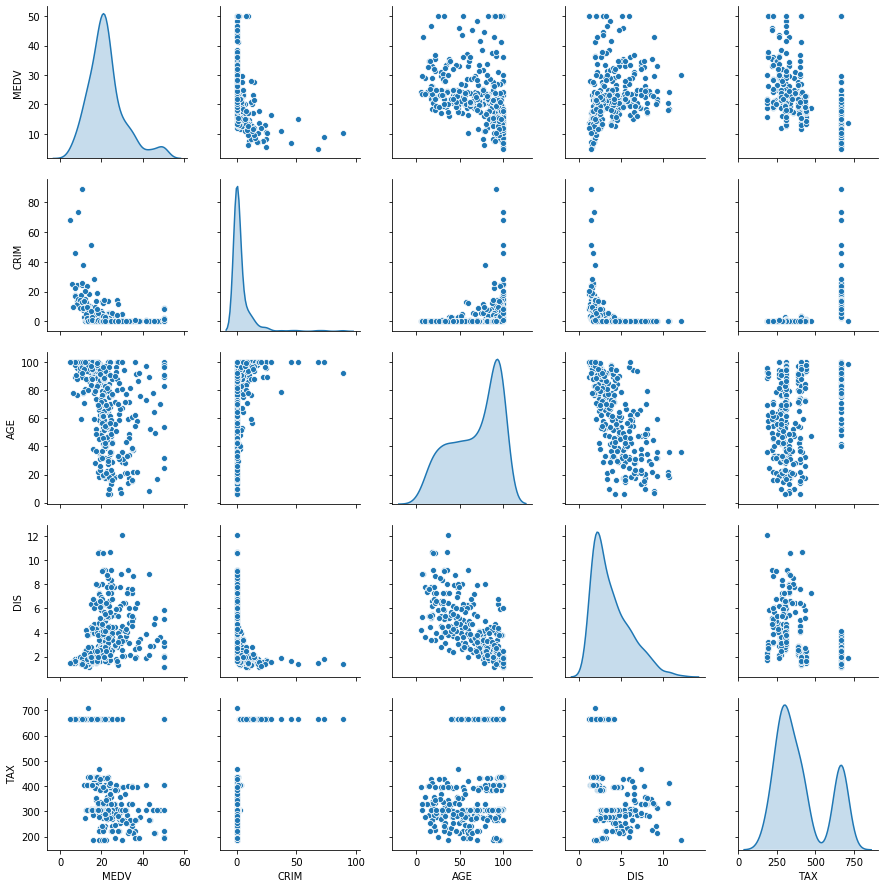Also look at overall statistics:

In :
train_stats = train_dataset.describe()
train_stats.pop("MEDV")
train_stats = train_stats.transpose()
train_stats

Out:
count mean std min 25% 50% 75% max
CRIM 354.0 3.767375 9.418497 0.00906 0.082757 0.274475 3.077295 88.9762
ZN 354.0 11.079096 23.070178 0.00000 0.000000 0.000000 12.500000 95.0000
INDUS 354.0 11.185254 6.646944 0.74000 5.860000 9.795000 18.100000 27.7400
CHAS 354.0 0.070621 0.256554 0.00000 0.000000 0.000000 0.000000 1.0000
NOX 354.0 0.554098 0.115748 0.38500 0.453000 0.538000 0.624000 0.8710
RM 354.0 6.265791 0.699380 3.56100 5.878250 6.175000 6.605500 8.7800
AGE 354.0 68.057627 27.953167 6.00000 45.100000 76.500000 93.750000 100.0000
DIS 354.0 3.844439 2.187514 1.12960 2.073700 3.207450 5.214600 12.1265
RAD 354.0 9.440678 8.569207 1.00000 4.000000 5.000000 20.000000 24.0000
TAX 354.0 407.500000 162.296676 187.00000 287.000000 337.000000 666.000000 711.0000
PTRATIO 354.0 18.461299 2.149735 12.60000 17.325000 18.850000 20.200000 22.0000
B 354.0 352.720650 95.764288 2.60000 373.852500 390.945000 396.225000 396.9000
LSTAT 354.0 12.614011 7.020224 1.73000 7.347500 11.185000 16.635000 37.9700

### Split features from labels¶

Separate the target value, or "label", from the features. This label is the value that you will train the model to predict.

In :
train_labels = train_dataset.pop('MEDV')
test_labels = test_dataset.pop('MEDV')


### Normalize the data¶

Look again at the train_stats block above and note how different the ranges of each feature are.

It is good practice to normalize features that use different scales and ranges. Although the model might converge without feature normalization, it makes training more difficult, and it makes the resulting model dependent on the choice of units used in the input.

Note: Although we intentionally generate these statistics from only the training dataset, these statistics will also be used to normalize the test dataset. We need to do that to project the test dataset into the same distribution that the model has been trained on.

In :
def norm(x):
return (x - train_stats['mean']) / train_stats['std']
normed_train_data = norm(train_dataset)
normed_test_data = norm(test_dataset)


This normalized data is what we will use to train the model.

Caution: The statistics used to normalize the inputs here (mean and standard deviation) need to be applied to any other data that is fed to the model, along with the one-hot encoding that we did earlier. That includes the test set as well as live data when the model is used in production.

### The Neural Network Model¶

#### > Build the model¶

Let's build our model. Here, we'll use a Sequential model with two densely connected hidden layers, and an output layer that returns a single, continuous value. The model building steps are wrapped in a function, build_model, since we'll create a second model, later on.

In :
def build_model():
model = keras.Sequential([
layers.Dense(64, activation='relu', input_shape=[len(train_dataset.keys())]),
layers.Dense(64, activation='relu'),
layers.Dense(1)
])

optimizer = tf.keras.optimizers.RMSprop(0.001)

model.compile(loss='mse',
optimizer=optimizer,
metrics=['mae', 'mse'])
return model

In :
model = build_model();


#### Inspect the model¶

Use the .summary method to print a simple description of the model

In :
model.summary()

Model: "sequential_1"
_________________________________________________________________
Layer (type)                 Output Shape              Param #
=================================================================
dense_3 (Dense)              (None, 64)                896
_________________________________________________________________
dense_4 (Dense)              (None, 64)                4160
_________________________________________________________________
dense_5 (Dense)              (None, 1)                 65
=================================================================
Total params: 5,121
Trainable params: 5,121
Non-trainable params: 0
_________________________________________________________________


Now try out the model. Take a batch of 10 examples from the training data and call model.predict on it.

In :
example_batch = normed_train_data[:10]
example_result = model.predict(example_batch)
example_result

Out:
array([[-0.19954365],
[ 0.17270532],
[-0.40494272],
[ 0.4093608 ],
[-0.03134198],
[-0.2191115 ],
[ 0.11227794],
[-0.36424917],
[-0.566972  ],
[ 0.08759232]], dtype=float32)

It seems to be working, and it produces a result of the expected shape and type.

### Train the model¶

Train the model for 1000 epochs, and record the training and validation accuracy in the history object.

Note the validation_split set to use 20% of the training data as validation set and the remainder as calibration. Important to note that this is separate from the testing data that we do not touch in the model-training.

In :
# Display training progress by printing a single dot for each completed epoch
class PrintDot(keras.callbacks.Callback):
def on_epoch_end(self, epoch, logs):
if epoch % 100 == 0: print('')
print('.', end='')

EPOCHS = 1000

history = model.fit(
normed_train_data, train_labels,
epochs=EPOCHS, validation_split = 0.2, verbose=0,
callbacks=[PrintDot()])

....................................................................................................
....................................................................................................
....................................................................................................
....................................................................................................
....................................................................................................
....................................................................................................
....................................................................................................
....................................................................................................
....................................................................................................
....................................................................................................

Visualize the model's training progress using the stats stored in the history object.

In :
hist = pd.DataFrame(history.history)
hist['epoch'] = history.epoch
hist.tail()

Out:
loss mean_absolute_error mean_squared_error val_loss val_mean_absolute_error val_mean_squared_error epoch
995 0.562552 0.524458 0.562552 15.975168 2.738591 15.975168 995
996 0.541612 0.523853 0.541612 14.937943 2.667974 14.937943 996
997 0.643346 0.605599 0.643346 14.817849 2.660901 14.817849 997
998 0.448284 0.459243 0.448284 16.806302 2.786597 16.806303 998
999 0.627310 0.584064 0.627310 14.821295 2.628259 14.821296 999
In :
def plot_history(history):
hist = pd.DataFrame(history.history)
hist['epoch'] = history.epoch

plt.figure()
plt.xlabel('Epoch')
plt.ylabel('Mean Abs Error [MEDV]')
plt.plot(hist['epoch'], hist['mean_absolute_error'],
label='Train Error')
plt.plot(hist['epoch'], hist['val_mean_absolute_error'],
label = 'Val Error')
plt.ylim([0,5])
plt.legend()

plt.figure()
plt.xlabel('Epoch')
plt.ylabel('Mean Square Error [$MEDV^2$]')
plt.plot(hist['epoch'], hist['mean_squared_error'],
label='Train Error')
plt.plot(hist['epoch'], hist['val_mean_squared_error'],
label = 'Val Error')
plt.ylim([0,20])
plt.legend()
plt.show()

plot_history(history)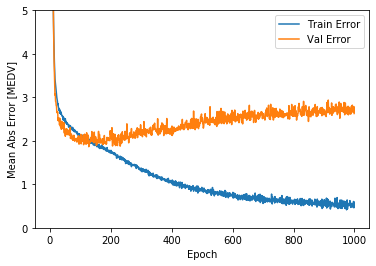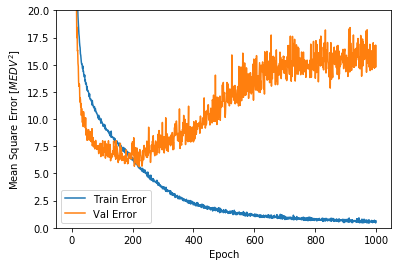This graph shows little improvement, or actually a fairly severe degradation in the validation error after about 100 epochs. Let's update the model.fit call to automatically stop training when the validation score doesn't improve. We'll use an EarlyStopping callback that tests a training condition for every epoch. If a set amount of epochs elapses without showing improvement, then automatically stop the training.

In :
model = build_model()

# The patience parameter is the amount of epochs to check for improvement
early_stop = keras.callbacks.EarlyStopping(monitor='val_loss', patience=10)

history = model.fit(normed_train_data, train_labels, epochs=EPOCHS,
validation_split = 0.2, verbose=0, callbacks=[early_stop, PrintDot()])

....................................................................................................
...........................

Let's re-plot the history to hopefully see the model training stopping before things get worse for the validation data.

In :
plot_history(history)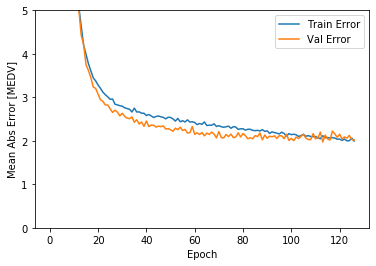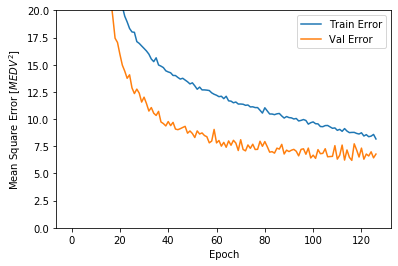The graph shows that on the validation set, the average error is usually around +/- 2 MEDV (or +/- 2,000 dollars from the true median value of owner-occupied homes). This is pretty good! And as we shall soon see, much better than the linear regression model!

Let's see how well the model generalizes by using the test set, which we did not use at all when training the model. This tells us how well we can expect the model to predict when we use it in the real world.

In :
loss, mae, mse = model.evaluate(normed_test_data, test_labels, verbose=2)

print("Testing set Mean Abs Error: {:5.2f} MEDV".format(mae))

152/152 - 0s - loss: 12.1545 - mean_absolute_error: 2.4443 - mean_squared_error: 12.1545
Testing set Mean Abs Error:  2.44 MEDV


### Make predictions¶

Finally, we predict MEDV values using data in the testing set (and also training, which we will use in the next step to compute more error metrics):

In :
test_predictions = model.predict(normed_test_data).flatten()
train_predictions = model.predict(normed_train_data).flatten()

plt.scatter(test_labels, test_predictions)
plt.xlabel('True Values [MEDV]')
plt.ylabel('Predictions [MEDV]')
plt.axis('equal')
plt.axis('square')
plt.xlim([0,plt.xlim()])
plt.ylim([0,plt.ylim()])
_ = plt.plot([-100, 100], [-100, 100])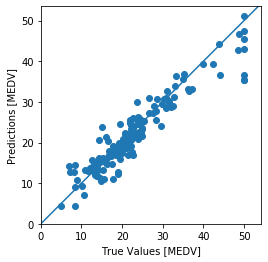### Error analysis¶

The graph above looks pretty, pretty, pretty, good! (pardon the Curb reference!). To get more than a visual understanding of the error, let's compute some error metrics.

We start with developing an empirical distribution of the error term (this is a very useful piece of code!). If you compare this to the distribution you saw in the OLS example, you can spot that this model definitely has a better performance.

In :
error = test_predictions - test_labels
plt.hist(error, bins = 25)
plt.xlabel("Prediction Error [MEDV]")
_ = plt.ylabel("Count")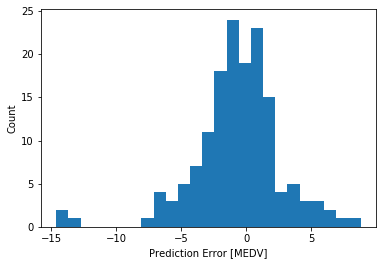Just like we did in the OLS example, let's calculate the mean-squared error, mean absolute error, and the r-squared error on training and testing. This is useful as we can see the extent to which performance degrades from training to testing data (note: we expect this degradation).

In :
from sklearn.metrics import mean_squared_error, mean_absolute_error, r2_score
mse = mean_squared_error(test_labels, test_predictions)
print('Mean Squared Error: ',mse)
mae = mean_absolute_error(test_labels, test_predictions)
print('Mean Absolute Error: ',mae)
rsq = r2_score(train_labels,train_predictions) #R-Squared on the training data
print('R-square, Training: ',rsq)
rsq = r2_score(test_labels,test_predictions) #R-Squared on the testing data
print('R-square, Testing: ',rsq)

Mean Squared Error:  12.154508327296316
Mean Absolute Error:  2.4443242976540014
R-square, Training:  0.8929076880994002
R-square, Testing:  0.877196340267011


If you compare the error statistics above to the OLS example using Boston House Price data, you will see that the metrics are vastly better! An average error of +/- \$2,400 vs \\$3,500! Also, the R-square value is much better at 88% on training data vs. 69%. You can perform minor modifications of the training routine (e.g. more epochs, different activation function, etc.) to get even better results.

This notebook shows how tremendously powerful the tensor flow neural network technique can be to craft highly predictive models as compared to regression. Of course, regression models are easier to communicate given they are much more broadly known and understood. The perfect model for your application will depend not just on the predictive accuracy, but also on how effective it is given your application and target audience.

### Feedback¶

If you have ideas on how to improve this post, please let me know: https://predictivemodeler.com/feedback/